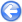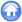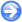Author
Stéphane Popinet
Command
sh geo.sh geo.gfs
Version
100323
Required files
geo.sh geo.gfv e.ref
Running time
2 minutes 46 seconds

We consider the geostrophic adjustment problem studied by Dupont  and Le Roux et al . A Gaussian bump

η ( xy ) = η0 e
−
 x2 + y2 R2

is initialised in a 1000×1000 km, 1000 m deep square basin. A reduced gravity g = 0.01 m/s2 is used to approximate a 10 m-thick stratified surface layer. On an f-plane the corresponding geostrophic velocities are given by

u ( xy )=
 2 g η0 y f0 R2
e
−
 x2 + y2 R2

,
v ( xy )=
−
 2 g η0 x f0 R2
e
−
 x2 + y2 R2

,

where f0 is the Coriolis parameter. Following Dupont we set f0 = 1.0285 × 10− 4 s− 1, R = 100 km, η0 = 599.5 m which gives a maximum geostrophic velocity of 0.5 m/s.

In the context of the linearised shallow-water equations, the geostrophic balance is an exact solution which should be preserved by the numerical method. In practice, this would require an exact numerical balance between terms computed very differently: the pressure gradient and the Coriolis terms in the momentum equation. If this numerical balance is not exact, the numerical solution will adjust toward numerical equilibrium through the emission of gravity-wave noise which should not affect the stability of the solution. This problem is thus a good test of both the overall accuracy of the numerical scheme and its stability properties when dealing with inertia–gravity waves. We note in particular that a standard A-grid discretisation would develop a strong computational-mode instability in this case. Also, as studied by Leroux et al, an inappropriate choice of finite-element basis functions will result in growing gravity-wave noise.

 Figure 128: Evolution of the maximum error on the surface height for the geostrophic adjustment problem.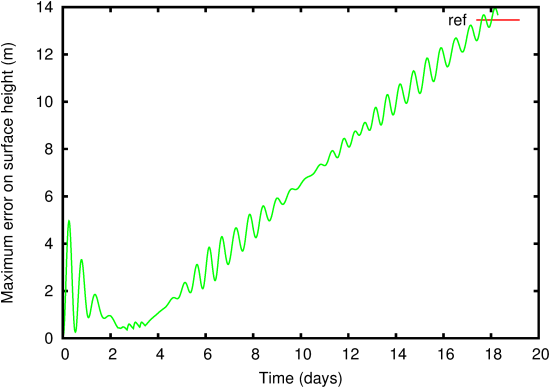Figure 129: Evolution of the surface-height error field. (a) t =1.157 days, (b) t = 2.315 days, (c) t =3.472 days, (d) t =4.630 days, (e) t =17.361 days.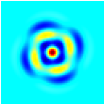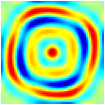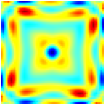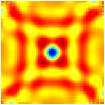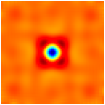(a) (b) (c) (d) (e)

Figures 128 and 129 summarise the results obtained when running the geostrophic adjustment problem on a 64 × 64 uniform grid with a timestep Δ t = 1000 s. The maximum error on the height field (Figure 128) is small even after 18 days. After a strong initial transient corresponding to the emission of gravity waves, the error reaches a minimum at day 3 and then slowly grows with time with modulations due to the reflexions of the initial gravity waves on the domain boundaries. As illustrated on figure 129, this growth is not due to any instability of the solution but to the slow decrease of the maximum amplitude of the Gaussian bump due to numerical energy dissipation.

#### 11.1.1PASS: Geostrophic adjustment on a beta-plane

Author
Stéphane Popinet
Command
sh beta.sh beta.gfs
Version
100323
Required files
beta.sh c dlw lls pzm llw energy.ref energy-nonlinear.ref
Running time
6 minutes 42 seconds

Same as before but a beta-plane, f = f0 + β y is used and the advection terms are included in the momentum equation. No explicit dissipation is added. As in  we chose β = 1.607 × 10− 11 m− 1s− 1. The geostrophic eddy moves slowly westward through the emission of Rossby waves and southward due to the non-linear advection term. The resulting evolution of the total energy is shown on figure 130. For our method, the slow decrease in the total energy is due both to the dissipation of potential energy induced by the approximate projection operator and to the dissipative properties of the BCG upwind advection scheme. Another run with the advection terms switched off (figure 130, green curve) confirms that the dissipation induced by the approximate projection operator dominates the total dissipation. The results however compare favourably with the finite-element formulations tested by Dupont which all show significantly larger energy dissipation.

 Figure 130: Evolution of the total energy for the non-linear geostrophic adjustment problem. The C-grid model is based on Sadourny [] and implemented by Dupont . The finite-element formulations are those studied by Dupont. LW: Lynch and Werner , LLS: Le Roux et al , PZM: Peraire et al .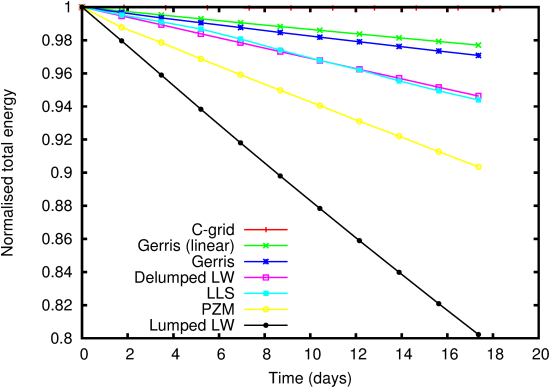#### 11.1.2PASS: Geostrophic adjustment with Saint-Venant

Author
Stéphane Popinet
Command
sh geo.sh river.gfs
Version
130112
Required files
geo.sh geo.gfv e.ref
Running time
42 seconds

Same test but using the (fully nonlinear) Saint-Venant solver.

 Figure 131: Evolution of the maximum error on the surface height for the geostrophic adjustment problem.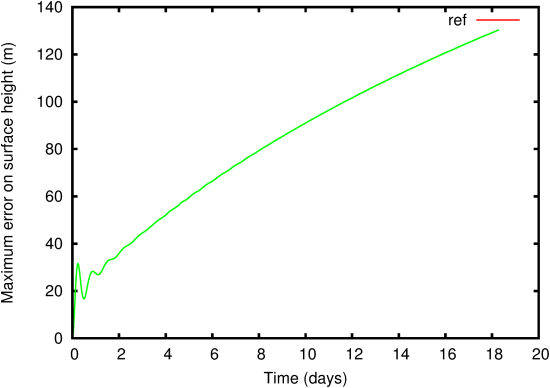Figure 132: Evolution of the surface-height error field. (a) t =1.157 days, (b) t = 2.315 days, (c) t =3.472 days, (d) t =4.630 days, (e) t =17.361 days.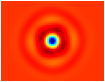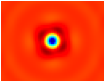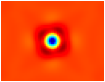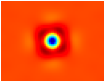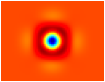(a) (b) (c) (d) (e)